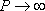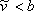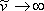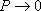# 9.2: The Cubic Behavior

Let us see what we have accomplished thus far. First, remember that we are not satisfied with the fact that the ideal gas law is not able to predict the discontinuity of Figure 6.3, which corresponds to the condensation that an all-gas pure substance undergoes during isothermal compression.The isotherm that we get from the ideal model was shown in Figure 6.2. Condensation, as we know, is to be expected at some point when you isothermally compress any pure gas while below its critical conditions (T < Tc). This lack of conformance to actual behavior led us to realize that the ideal model is quantitatively and qualitatively wrong at high pressures. To remove the weaknesses of the ideal model, we recall that vdW, in developing his equation of state (equation 7.11), introduced the concepts of co-volume and the attraction term.

Now, we wonder, what did he really accomplish? Are we now able to predict the condensation phenomena? Are these “new” cubic-type EOS capable of showing where such a discontinuity occurs? So far, we have not seen what a cubic isotherm looks like. Let us plot the cubic isotherm for conditions below critical (T < Tc), superimpose it on Figure 6.3, and see what we get.Figure 9.1: P-v Isotherm for a cubic EOS

Figure 9.1 shows a typical cubic behavior. That is, around condensation conditions, the equation of state presents an S-shaped curve. This should not come as a surprise; because the equation is cubic in volume, it will provide three roots for volume, and hence the S-shaped cubic feature. For the sake of our discussion, we have shown the gas and liquid branches of the cubic equation lying directly on top of the experimental ones. This kind of matching is the “ultimate goal” of any cubic EOS, but in reality, the matching is not this good — especially for the case of the original vdW EOS, as we will discuss later.

The cubic behavior is bounded by the two extremes of real fluid behavior, given by the zero pressure and infinite pressure limits. On one hand, it is clear from equation (7.11b) that we have a singularity at, where “b” represents the co-volume, or physical space that molecules themselves occupy. This singularity conveniently creates an asymptotic behavior (high pressure asymptote) of the cubic equation liquid branch, by whichas. Recall from our previous discussions that predictions forare meaningless. Accordingly, we need an infinite amount of pressure if we are to compress a fluid to the extent that no free space is available among molecules (). On the other hand, it is clear from vdW EOS (equation 7.11a) that as(), the cubic EOS collapses to the ideal EOS (equation 7.6). At this low pressure limit, vdW
corrections to the ideal model become inconsequential (,).

Mathematically, this is the low-pressure asymptote of the cubic-equation gas branch by whichas.

Let us see how “good” the cubic behavior is for the ideal-gas model. Refer to Figure 9.2, where we have superimposed the ideal gas isotherm of Figure 6.2 on the cubic EOS behavior.Figure 9.2: P-v Isotherms for the ideal gas EOS and cubic EOS

A look at Figure 9.2 helps us confirm that the cubic equation of state collapses to the prediction of the ideal gas model at reduced pressure — i.e., they share the same low-pressure asymptote. This is to be expected, since the assumptions underlying the ideal model are satisfied. As we recall, these assumptions are that the attractive forces between molecules are very weak and that the physical volume of the molecules can be disregarded when compared to the total volume of the container. It is worthy to note that the high pressure asymptote is not the same for both models; as,as for the cubic model whilefor the ideal model. The latter is a direct consequence of neglecting the molecular volume in the ideal model.

## Contributors

• Prof. Michael Adewumi (The Pennsylvania State University). Some or all of the content of this module was taken from Penn State's College of Earth and Mineral Sciences' OER Initiative.